This article was published as a part of the Data Science Blogathon

## Introduction

Decision Trees which are supervised Machine Learning Algorithms are one of the simplest algorithms that can be used for both regression and classification problems.

Therefore it becomes necessary for every aspiring Data Scientist and Machine Learning Engineer to have a good knowledge of Decision Trees.

In this article, we will discuss the most important questions on the Decision Trees which is helpful to get you a clear understanding of the techniques, and also for Data Science Interviews, which covers its very fundamental level to complex concepts.

## 1. What is the Decision Tree Algorithm?

A Decision Tree is a supervised machine learning algorithm that can be used for both Regression and Classification problem statements. It divides the complete dataset into smaller subsets while at the same time an associated Decision Tree is incrementally developed.

The final output of the Decision Trees is a Tree having Decision nodes and leaf nodes. A Decision Tree can operate on both categorical and numerical data.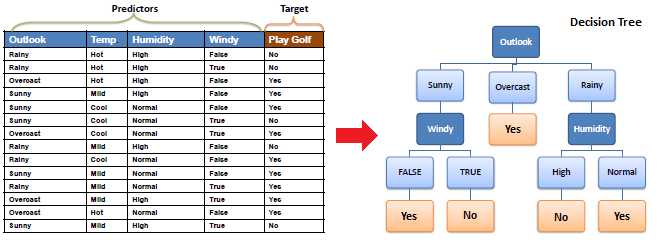## 2. List down some popular algorithms used for deriving Decision Trees along with their attribute selection measures.

Some of the popular algorithms used for constructing decision trees are:

1. ID3 (Iterative Dichotomiser): Uses Information Gain as attribute selection measure.

2. C4.5 (Successor of ID3):  Uses Gain Ratio as attribute selection measure.

3. CART (Classification and Regression Trees) – Uses Gini Index as attribute selection measure.

## 3. Explain the CART Algorithm for Decision Trees.

The CART stands for Classification and Regression Trees is a greedy algorithm that greedily searches for an optimum split at the top level, then repeats the same process at each of the subsequent levels.

Moreover, it does verify whether the split will lead to the lowest impurity or not as well as the solution provided by the greedy algorithm is not guaranteed to be optimal, it often produces a solution that’s reasonably good since finding the optimal Tree is an NP-Complete problem that requires exponential time complexity.

As a result, it makes the problem intractable even for small training sets. This is why we must go for a “reasonably good” solution instead of an optimal solution.

## 4. List down the attribute selection measures used by the ID3 algorithm to construct a Decision Tree.

The most widely used algorithm for building a Decision Tree is called ID3. ID3 uses Entropy and Information Gain as attribute selection measures to construct a Decision Tree.

1. Entropy:  A Decision Tree is built top-down from a root node and involves the partitioning of data into homogeneous subsets. To check the homogeneity of a sample, ID3 uses entropy. Therefore, entropy is zero when the sample is completely homogeneous, and entropy of one when the sample is equally divided between different classes.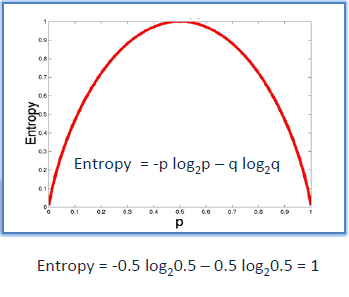2. Information Gain:  Information Gain is based on the decrease in entropy after splitting a dataset based on an attribute. The meaning of constructing a Decision Tree is all about finding the attributes having the highest information gain.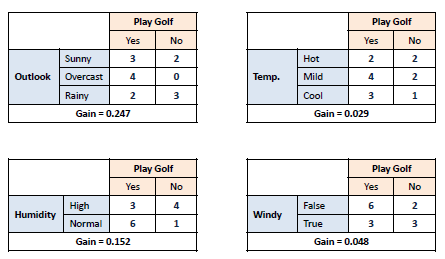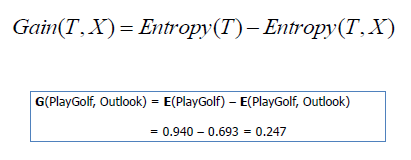## 5. Briefly explain the properties of Gini Impurity.

Let X (discrete random variable) takes values y₊ and y₋ (two classes). Now, let’s consider the different cases:

Case- 1:  When 100% observations belong to y₊ . Then, the Gini impurity of the system would be: –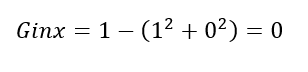Case- 2:  When 50% observations belong to y₊ . Then, the Gini impurity of the system would be: –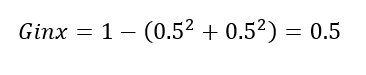Case- 3:  When 0% observations belong to y₊ . Then, the Gini impurity of the system would be: –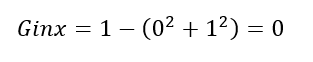After observing all these cases, the graph of Gini impurity w.r.t to y₊ would come out to be: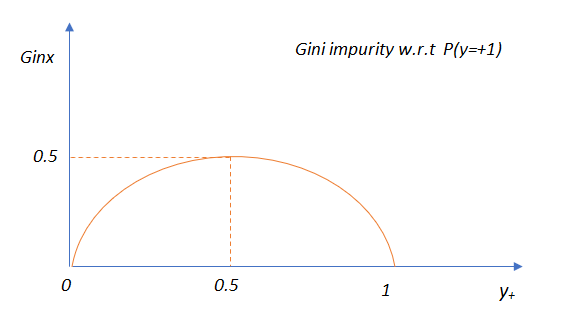## 6. Explain the difference between the CART and ID3 Algorithms.

The CART algorithm produces only binary Trees: non-leaf nodes always have two children (i.e., questions only have yes/no answers).

On the contrary, other Tree algorithms such as ID3 can produce Decision Trees with nodes having more than two children.

## 7. Which should be preferred among Gini impurity and Entropy?

In reality, most of the time it does not make a big difference: they lead to almost similar Trees. Gini impurity is a good default while implementing in sklearn since it is slightly faster to compute.

However, when they work in a different way, then Gini impurity tends to isolate the most frequent class in its own branch of the Tree, while entropy tends to produce slightly more balanced Trees.

## 8. List down the different types of nodes in Decision Trees.

The Decision Tree consists of the following different types of nodes:

1. Root node: It is the top-most node of the Tree from where the Tree starts.

2. Decision nodes: One or more Decision nodes that result in the splitting of data into multiple data segments and our main goal is to have the children nodes with maximum homogeneity or purity.

3. Leaf nodes: These nodes represent the data section having the highest homogeneity.

9. What do you understand about Information Gain? Also, explain the mathematical formulation associated with it.

Information gain is the difference between the entropy of a data segment before the split and after the split i.e, reduction in impurity due to the selection of an attribute.

Some points keep in mind about information gain:

• The high difference represents high information gain.
• Higher the difference implies the lower entropy of all data segments resulting from the split.
• Thus, the higher the difference, the higher the information gain, and the better the feature used for the split.

Mathematically, the information gain can be computed by the equation as follows:

Information Gain = E(S1) – E(S2)

E(S1) denotes the entropy of data belonging to the node before the split.

E(S2) denotes the weighted summation of the entropy of children nodes by considering the weights as the proportion of data instances falling in specific children nodes.

10. Do we require Feature Scaling for Decision Trees? Explain.

Decision Trees are mainly intuitive, easy to interpret as well as require less data preparation. In fact, they don’t require feature scaling or centering(standardization) at all. Such models are often called white-box models.

Decision Trees provide simple classification rules based on if and else statements which can even be applied manually if need be.

For Example, Flower classification for the Iris dataset.

11. What are the disadvantages of Information Gain?

Information gain is defined as the reduction in entropy due to the selection of a particular attribute. Information gain biases the Decision Tree against considering attributes with a large number of distinct values which might lead to overfitting.

In order to solve this problem, the Information Gain Ratio is used.

12. List down the problem domains in which Decision Trees are most suitable.

Decision Trees are suitable for the following cases:

1. Decision Trees are most suitable for tabular data.

2. The outputs are discrete.

3. Explanations for Decisions are required.

4. The training data may contain errors, noisy data(outliers).

5. The training data may contain missing feature values.

13. Explain the time and space complexity of training and testing in the case of a Decision Tree.

Time and Space complexity for Training:

In the training stage for features (dimensions) in the dataset, we sort the data which takes O(n log n) time following which we traverse the data points to find the right threshold which takes O(n) time. Subsequently, for d dimensions, the total time complexity would be: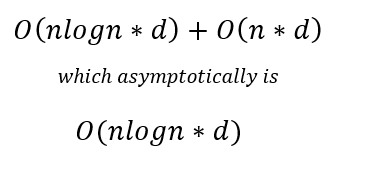Usually while training a decision tree we identify the nodes which are typically stored in the form of if-else statements due to which training space complexity is O(nodes).

Time and Space Complexity for Testing:

Moreover, the testing time complexity is O(depth) as we have to traverse from the root to a leaf node of the decision tree i.e., testing space complexity is O(nodes).

14. If it takes one hour to train a Decision Tree on a training set containing 1 million instances, roughly how much time will it take to train another Decision Tree on a training set containing 10 million instances?

As we know that the computational complexity of training a Decision Tree is given by O(n × m log(m)). So, when we multiplied the size of the training set by 10, then the training time will be multiplied by some factor, say K.

Now, we have to determine the value of K. To finds K, divide the complexity of both:

K = (n × 10m × log(10m)) / (n × m × log(m)) = 10 × log(10m) / log(m)

For 10 million instances i.e., m = 106, then we get the value of K ≈ 11.7.

Therefore, we can expect the training time to be roughly 11.7 hours.

15. How does a Decision Tree handle missing attribute values?

Decision Trees handle missing values in the following ways:

• Fill the missing attribute value by the most common value of that attribute.
• Fill the missing value by assigning a probability to each of the possible values of the attribute based on other samples.

16. How does a Decision Tree handle continuous(numerical) features?

Decision Trees handle continuous features by converting these continuous features to a threshold-based boolean feature.

To decide The threshold value, we use the concept of Information Gain, choosing that threshold that maximizes the information gain.

17. What is the Inductive Bias of Decision Trees?

The ID3 algorithm preferred Shorter Trees over longer Trees. In Decision Trees, attributes having high information gain are placed close to the root are preferred over those that do not.

18. Explain Feature Selection using the Information Gain/Entropy Technique.

The goal of the feature selection while building a Decision Tree is to select features or attributes (Decision nodes) which lead to a split in children nodes whose combined entropy adds up to lower entropy than the entropy value of the data segment before the split. This implies higher information gain.

19. Compare the different attribute selection measures.

The three measures, in general, returns good results, but:

1. Information Gain: It is biased towards multivalued attributes

2. Gain ratio: It prefers unbalanced splits in which one data segment is much smaller than the other segment.

3. Gini Index: It is biased to multivalued attributes, has difficulty when the number of classes is large, tends to favor tests that result in equal-sized partitions and purity in both partitions.

20. Does the Gini Impurity of a node lower or greater than that of its parent. Comment whether it is generally lower/greater, or always lower/greater?

A node’s Gini impurity is generally lower than that of its parent as the CART training algorithm cost function splits each of the nodes in a way that minimizes the weighted sum of its children’s Gini impurities. However, sometimes it is also possible for a node to have a higher Gini impurity than its parent but in such cases, the increase is more than compensated by a decrease in the other child’s impurity.

For better understanding we consider the following Example:

Consider a node containing four samples of class A and one sample of class B.

Then, its Gini impurity is calculated as 1 – (1/5)2 – (4/5)2= 0.32

Now suppose the dataset is one-dimensional and the instances are arranged in the manner: A, B, A, A, A. We can verify that the algorithm will split this node after the second instance, producing one child node with instances A, B, and the other child node with instances A, A, A.

Then, the first child node’s Gini impurity is 1 – (1/2)2 – (1/2)2 = 0.5, which is higher than its parent’s. This is compensated for by the fact that the other node is pure, so its overall weighted Gini impurity is 2/5 × 0.5 + 3/5 × 0 = 0.2, which is lower than the parent’s Gini impurity.

21. Why do we require Pruning in Decision Trees? Explain.

After we create a Decision Tree we observe that most of the time the leaf nodes have very high homogeneity i.e., properly classified data. However, this also leads to overfitting. Moreover, if enough partitioning is not carried out then it would lead to underfitting.

Hence the major challenge that arises is to find the optimal trees which result in the appropriate classification having acceptable accuracy. So to cater to those problems we first make the decision tree and then use the error rates to appropriately prune the trees.

22. Are Decision Trees affected by the outliers? Explain.

Decision Trees are not sensitive to noisy data or outliers since, extreme values or outliers, never cause much reduction in Residual Sum of Squares(RSS), because they are never involved in the split.

## 23. What do you understand by Pruning in a Decision Tree?

When we remove sub-nodes of a Decision node, this process is called pruning or the opposite process of splitting. The two techniques which are widely used for pruning are- Post and Pre Pruning.

Post Pruning:

• This type of pruning is used after the construction of the Decision Tree.
• This technique is used when the Decision Tree will have a very large depth and will show the overfitting of the model.
• It is also known as backward pruning.
• This technique is used when we have an infinitely grown Decision Tree.

Pre Pruning:

• This technique is used before the construction of the Decision Tree.
• Pre-Pruning can be done using Hyperparameter tuning.
• Overcome the overfitting issue.

24. List down the advantages of the Decision Trees.

1. Clear Visualization:  This algorithm is simple to understand, interpret and visualize as the idea is mostly used in our daily lives. The output of a Decision Tree can be easily interpreted by humans.

2. Simple and easy to understand: Decision Tree works in the same manner as simple if-else statements which are very easy to understand.

3. This can be used for both classification and regression problems.

4. Decision Trees can handle both continuous and categorical variables.

5. No feature scaling required: There is no requirement of feature scaling techniques such as standardization and normalization in the case of Decision Tree as it uses a rule-based approach instead of calculation of distances.

6. Handles nonlinear parameters efficiently: Unlike curve-based algorithms, the performance of decision trees can’t be affected by the Non-linear parameters. So, if there is high non-linearity present between the independent variables, Decision Trees may outperform as compared to other curve-based algorithms.

7. Decision Tree can automatically handle missing values.

8. Decision Tree handles the outliers automatically, hence they are usually robust to outliers.

9. Less Training Period: The training period of decision trees is less as compared to ensemble techniques like Random Forest because it generates only one Tree unlike the forest of trees in the Random Forest.

25. List out the disadvantages of the Decision Trees.

1. Overfitting: This is the major problem associated with the Decision Trees. It generally leads to overfitting of the data which ultimately leads to wrong predictions for testing data points. it keeps generating new nodes in order to fit the data including even noisy data and ultimately the Tree becomes too complex to interpret. In this way, it loses its generalization capabilities. Therefore, it performs well on the training dataset but starts making a lot of mistakes on the test dataset.

2. High variance: As mentioned, a Decision Tree generally leads to the overfitting of data. Due to the overfitting, there is more likely a chance of high variance in the output which leads to many errors in the final predictions and shows high inaccuracy in the results. So, in order to achieve zero bias (overfitting), it leads to high variance due to the bias-variance tradeoff.

3. Unstable: When we add new data points it can lead to regeneration of the overall Tree. Therefore, all nodes need to be recalculated and reconstructed.

4. Not suitable for large datasets: If the data size is large, then one single Tree may grow complex and lead to overfitting. So in this case, we should use Random Forest instead, an ensemble technique of a single Decision Tree.

## End Notes

I hope you enjoyed the questions and were able to test your knowledge about Decision Trees.

If you liked this and want to know more, go visit my other articles on Data Science and Machine Learning by clicking on the Link

Something not mentioned or want to share your thoughts? Feel free to comment below And I’ll get back to you.

## Chirag Goyal

Currently, I am pursuing my Bachelor of Technology (B.Tech) in Computer Science and Engineering from the Indian Institute of Technology Jodhpur(IITJ). I am very enthusiastic about Machine learning, Deep Learning, and Artificial Intelligence.

The media shown in this article are not owned by Analytics Vidhya and is used at the Author’s discretion.